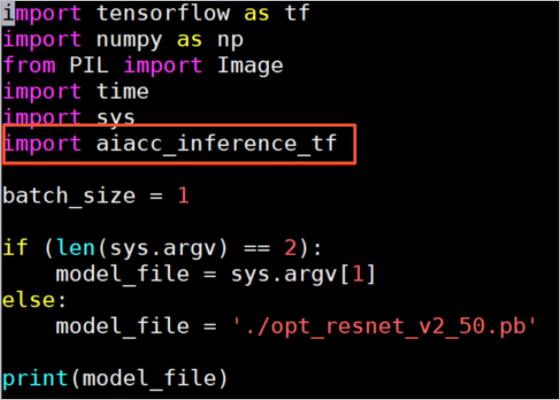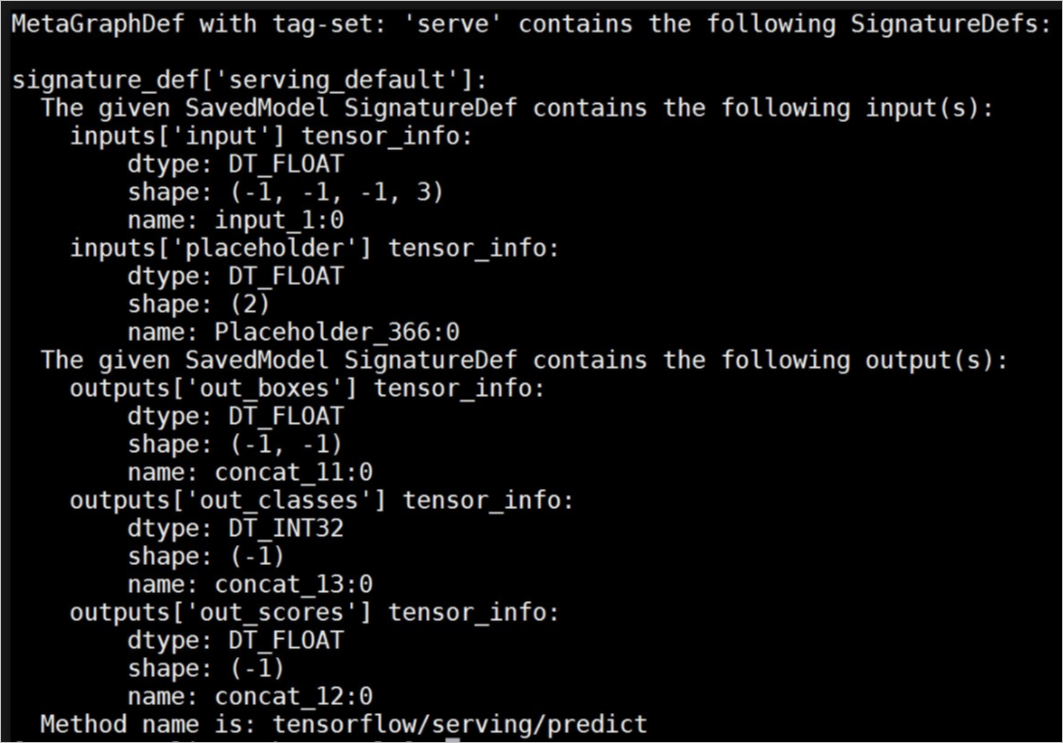To use AIACC-Inference in TensorFlow, you must call the interface of AIACC-Inference in TensorFlow to optimize models. This topic describes how to optimize a model and execute inference tasks.

## Background information

AIACC-Inference in TensorFlow supports models that are used to classify and detect images. To optimize a model, AIACC-Inference analyzes the computation graph of the model and fuses the compute nodes in the model. This reduces the number of compute nodes and improves the efficiency when the computation graph is executed. AIACC-Inference in TensorFlow supports optimized models which include but are not limited to:
• ResNet
• Inception V4
• SSD
• Faster-RCNN
• Yolo V3

AIACC-Inference in TensorFlow allows you to optimize the options of FP32 and FP16 precision models. Tensor Core hardware in NVIDIA Volta and Turing architectures improves the inference performance on V100 and T4 GPUs.

## Procedure

2. Call the interface optimization model.
The following description shows the interface of optimization models:
3. Perform inference tasks based on the optimized models.
Add the library of AIACC-Inference in TensorFlow to the code without the need to modify the code.
``import aiacc_inference_tf``
Note You cannot use different types of models. For example, models optimized on V100 GPUs cannot be used to perform inference tasks on T4 GPUs.## Input in the frozen graph format and output in the frozen graph format

Optimize the interface definition:
``optimize_tf_model(model_fname, output_names, output_model, batch_size, precision)``
Table 1. Parameter descriptions of optimize_tf_model
Parameter Type Description
graph_file String The file name of the original model. The formal parameter that corresponds to model_fname is related to the format. The frozen graph format corresponds to graph_file.
output_names String List The output node name of the original model.
output_model String The file name of the optimized model.
batch_size List The batch size used when an inference task is executed based on the model. You can specify multiple batch sizes, and use the batch sizes when the model is generated.
precision String The precision used when the model is optimized. Valid values:
• FP32
• FP16
Example of optimizing the Inception-v4 model:
``````import tensorflow as tf
from aiacc_inference_tf.libaiacc_inference_tf import *

graph_file = './inception_v4.pb'
output_names = ['classes', 'logits']
output_model = './opt_model.pb'
batch_size = [ 1, 2, 4, 8 ]
optimize_tf_model(graph_file, output_names, output_model, batch_size, 'FP16')``````

## Input in the frozen graph format and output in the SavedModel format

Optimize the interface definition:
``````optimize_tf_model_v2(model_fname, saved_model_dir,
input_names, output_names,
input_tensor_names, output_tensor_names,
signature_key,
batch_size, precision)``````
Table 2. Parameter descriptions of optimize_tf_model_v2
Parameter Type Description
graph_pb String The file name of the original model. The formal parameter that corresponds to model_fname is related to the format. The frozen graph format corresponds to graph_pb.
export_dir String The name of the Saved Model directory to which the optimized model belongs. The formal parameter that corresponds to saved_model_dir is related to the format. The SavedModel format corresponds to export_dir.
input_names String List The name of the input node that is specified by SignatureDef in Saved Model.
output_names String List The name of the output node that is specified by SignatureDef in Saved Model.
input_tensor_names String List The input node name of the original model.
output_tensor_names String List The output node name of the original model.
signature_key String The signature key of SignatureDef used by Saved Model.

If the value of this parameter is None, `serving_default` is used by default.

batch_size List The batch size used when an inference task is executed based on the model. You can specify multiple batch sizes, and use the batch sizes when the model is generated.
precision String The precision used when the model is optimized. Valid values:
• FP32
• FP16
Example of optimizing the YOLOv3 model:
``````import tensorflow as tf
from aiacc_inference_tf.libaiacc_inference_tf import *

export_dir = './saved_model/1'
graph_pb = './yolo.pb'
input_names = [ 'input', 'placeholder' ]
output_names = [ 'out_boxes', 'out_scores', 'out_classes' ]
input_tensor_names = ['input_1', 'Placeholder_366']
output_tensor_names = ['concat_11', 'concat_12', 'concat_13']
signature_key = 'predict'
batch_size = [ 1 ]
optimize_tf_model_v2(graph_pb, export_dir, input_names, output_names,
input_tensor_names, output_tensor_names, signature_key,  batch_size, 'FP16')``````
To view the information about the SavedModel model and the input and output information about SignatureDef, use saved_model_cli. Example:## Input in the SavedModel format and output in the SavedModel format

Optimize the interface definition:
``optimize_tf_saved_model(input_model_dir, saved_model_dir, signature_key, batch_size, precision)``
Table 3. Parameter descriptions of optimize_tf_saved_model
Parameter Type Description
input_dir String The name of the Saved Model directory to which the original model belongs. The formal parameter that corresponds to input_model_dir is related to the format. The SavedModel format corresponds to input_model_dir.
export_dir String The name of the Saved Model directory to which the optimized model belongs. The formal parameter that corresponds to saved_model_dir is related to the format. The SavedModel format corresponds to export_dir.
signature_key String The signature key of SignatureDef used by Saved Model.

If the value of this parameter is None, `serving_default` is used by default.

batch_size List The batch size used when an inference task is executed based on the model. You can specify multiple batch sizes, and use the batch sizes when the model is generated.
precision String The precision used when the model is optimized. Valid values:
• FP32
• FP16
Example of optimizing the ResNet model:
``````import tensorflow as tf
from aiacc_inference_tf.libaiacc_inference_tf import *

input_dir = './resnet_v2_fp32_savedmodel_NHWC/1538****'
export_dir = './saved_model/1'
signature_key  = 'predict'
batch_size  = [ 1 ]
optimize_tf_saved_model(input_dir, export_dir, signature_key,  batch_size, 'FP16')``````

## Keras model

If you build a model based on Keras, you can convert the Keras model to a TensorFlow model and use AIACC-Inference in TensorFlow to optimize the model. The conversion method from Keras to TensorFlow is related to how the specific model is implemented. To load the model, you must call the tensorflow.keras.models.load_model() operation.
• If the model can be loaded, an interface is provided to convert the Keras model to the TensorFlow model and optimize the model.
• Otherwise, you must manually convert the Keras model to the TensorFlow model, and use AIACC-Inference in TensorFlow to optimize the model.
Optimize the interface definition:
``optimize_keras_model(model_fname, output_model, batch_size, precision)``
Table 4. Parameter descriptions of optimize_keras_model
Parameter Type Description
graph_file String The file name of the original model. The formal parameter that corresponds to model_fname is related to the format. The frozen graph format corresponds to graph_file.
output_model String The file name of the optimized model.
batch_size List The batch size used when an inference task is executed based on the model. You can specify multiple batch sizes, and use the batch sizes when the model is generated.
precision String The precision used when the model is optimized. Valid values:
• FP32
• FP16
Example of optimizing the Keras H5 model:
``````import tensorflow as tf
from aiacc_inference_tf.libaiacc_inference_tf import *

graph_file = './model.h5'
output_model = './opt_model.pb'
batch_size = [ 1 ]
optimize_keras_model(graph_file, output_model, batch_size, 'FP16')``````

## NPU model

Optimize the interface definition:
``````convert_npu_saved_model(model_fname, saved_model_dir,
input_names, output_names,
input_tensor_names, output_tensor_names,
signature_key)``````
Note This interface is used to optimize the NPU model that is quantized. This interface supports input in the frozen graph format and output in the SavedModel format.
Table 5. Parameter descriptions of convert_npu_saved_model
Parameter Type Description
graph_file String The file name of the original model. The formal parameter that corresponds to model_fname is related to the format. The frozen graph format corresponds to graph_file.
export_dir String The name of the SavedModel directory to which the optimized model belongs. The formal parameter that corresponds to saved_model_dir is related to the format. The SavedModel format corresponds to export_dir.
input_names String List The name of the input node that is specified by SignatureDef in SavedModel.
output_names String List The name of the output node that is specified by SignatureDef in SavedModel.
input_tensor_names String List The input node name of the original model.
output_tensor_names String List The output node name of the original model.
signature_key String The signature key of SignatureDef used by SavedModel.

If the value of this parameter is None, `serving_default` is used by default.

## StyleGAN model

Optimize the interface definition:
``convert_graph_to_half(graph_file, output_names, output_model, keep_list = [])``
Note This interface is used to convert the precision of the StyleGAN model from FP32 to FP16, and accelerates the process by compressing the model.
Table 6. Parameter descriptions of convert_graph_to_half
Parameter Type Description
graph_file String The file name of the original model.
output_names String List The output node name of the original model.
output_model String The file name of the optimized model.
keep_list String List The node that has an precision of FP32, which needs to be maintained in the original model.
Example of optimizing the model:
``````from aiacc_inference_tf.libaiacc_inference_tf import *

graph_file = 'graph.pb'
output_names = ['Gs/_Run/concat']
output_model = 'opt_graph.pb'
convert_graph_to_half(graph_file, output_names, output_model)``````